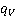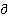### Create an Account

Home / Questions / Why can be equated with a state function if q is not a state function 2 Explain without ...

# Why can be equated with a state function if q is not a state function 2 Explain without using equations why HPT is generally small for a real gas

Why canbe equated with a state function if q is not a state function?

2. Explain without using equations why (H/P)T is generally small for a real gas.

Jun 10 2020 View more View LessSubscribe To Get Solution